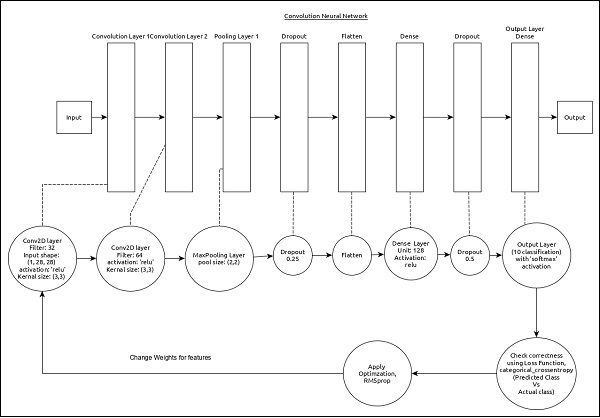# Keras - Convolution Neural Network

Let us modify the model from MPL to Convolution Neural Network (CNN) for our earlier digit identification problem.

CNN can be represented as below −The core features of the model are as follows −

• Input layer consists of (1, 8, 28) values.

• First layer, Conv2D consists of 32 filters and ‘relu’ activation function with kernel size, (3,3).

• Second layer, Conv2D consists of 64 filters and ‘relu’ activation function with kernel size, (3,3).

• Thrid layer, MaxPooling has pool size of (2, 2).

• Fifth layer, Flatten is used to flatten all its input into single dimension.

• Sixth layer, Dense consists of 128 neurons and ‘relu’ activation function.

• Seventh layer, Dropout has 0.5 as its value.

• Eighth and final layer consists of 10 neurons and ‘softmax’ activation function.

• Use categorical_crossentropy as loss function.

• Use accuracy as metrics.

• Use 128 as batch size.

• Use 20 as epochs.

Step 1 − Import the modules

Let us import the necessary modules.

```import keras
from keras.datasets import mnist
from keras.models import Sequential
from keras.layers import Dense, Dropout, Flatten
from keras.layers import Conv2D, MaxPooling2D
from keras import backend as K
import numpy as np
```

Let us import the mnist dataset.

```(x_train, y_train), (x_test, y_test) = mnist.load_data()
```

Step 3 − Process the data

Let us change the dataset according to our model, so that it can be feed into our model.

```img_rows, img_cols = 28, 28

if K.image_data_format() == 'channels_first':
x_train = x_train.reshape(x_train.shape, 1, img_rows, img_cols)
x_test = x_test.reshape(x_test.shape, 1, img_rows, img_cols)
input_shape = (1, img_rows, img_cols)
else:
x_train = x_train.reshape(x_train.shape, img_rows, img_cols, 1)
x_test = x_test.reshape(x_test.shape, img_rows, img_cols, 1)
input_shape = (img_rows, img_cols, 1)

x_train = x_train.astype('float32')
x_test = x_test.astype('float32')
x_train /= 255
x_test /= 255

y_train = keras.utils.to_categorical(y_train, 10)
y_test = keras.utils.to_categorical(y_test, 10)
```

The data processing is similar to MPL model except the shape of the input data and image format configuration.

Step 4 − Create the model

Let us create tha actual model.

```model = Sequential()
activation = 'relu', input_shape = input_shape))
model.add(Conv2D(64, (3, 3), activation = 'relu'))
```

Step 5 − Compile the model

Let us compile the model using selected loss function, optimizer and metrics.

```model.compile(loss = keras.losses.categorical_crossentropy,
```

Step 6 − Train the model

Let us train the model using fit() method.

```model.fit(
x_train, y_train,
batch_size = 128,
epochs = 12,
verbose = 1,
validation_data = (x_test, y_test)
)
```

Executing the application will output the below information −

```Train on 60000 samples, validate on 10000 samples Epoch 1/12
60000/60000 [==============================] - 84s 1ms/step - loss: 0.2687
- acc: 0.9173 - val_loss: 0.0549 - val_acc: 0.9827 Epoch 2/12
60000/60000 [==============================] - 86s 1ms/step - loss: 0.0899
- acc: 0.9737 - val_loss: 0.0452 - val_acc: 0.9845 Epoch 3/12
60000/60000 [==============================] - 83s 1ms/step - loss: 0.0666
- acc: 0.9804 - val_loss: 0.0362 - val_acc: 0.9879 Epoch 4/12
60000/60000 [==============================] - 81s 1ms/step - loss: 0.0564
- acc: 0.9830 - val_loss: 0.0336 - val_acc: 0.9890 Epoch 5/12
60000/60000 [==============================] - 86s 1ms/step - loss: 0.0472
- acc: 0.9861 - val_loss: 0.0312 - val_acc: 0.9901 Epoch 6/12
60000/60000 [==============================] - 83s 1ms/step - loss: 0.0414
- acc: 0.9877 - val_loss: 0.0306 - val_acc: 0.9902 Epoch 7/12
60000/60000 [==============================] - 89s 1ms/step - loss: 0.0375
-acc: 0.9883 - val_loss: 0.0281 - val_acc: 0.9906 Epoch 8/12
60000/60000 [==============================] - 91s 2ms/step - loss: 0.0339
- acc: 0.9893 - val_loss: 0.0280 - val_acc: 0.9912 Epoch 9/12
60000/60000 [==============================] - 89s 1ms/step - loss: 0.0325
- acc: 0.9901 - val_loss: 0.0260 - val_acc: 0.9909 Epoch 10/12
60000/60000 [==============================] - 89s 1ms/step - loss: 0.0284
- acc: 0.9910 - val_loss: 0.0250 - val_acc: 0.9919 Epoch 11/12
60000/60000 [==============================] - 86s 1ms/step - loss: 0.0287
- acc: 0.9907 - val_loss: 0.0264 - val_acc: 0.9916 Epoch 12/12
60000/60000 [==============================] - 86s 1ms/step - loss: 0.0265
- acc: 0.9920 - val_loss: 0.0249 - val_acc: 0.9922
```

Step 7 − Evaluate the model

Let us evaluate the model using test data.

```score = model.evaluate(x_test, y_test, verbose = 0)

print('Test loss:', score)
print('Test accuracy:', score)
```

Executing the above code will output the below information −

```Test loss: 0.024936060590433316
Test accuracy: 0.9922
```

The test accuracy is 99.22%. We have created a best model to identify the handwriting digits.

Step 8 − Predict

Finally, predict the digit from images as below −

```pred = model.predict(x_test)
pred = np.argmax(pred, axis = 1)[:5]
label = np.argmax(y_test,axis = 1)[:5]

print(pred)
print(label)
```

The output of the above application is as follows −

```[7 2 1 0 4]
[7 2 1 0 4]
```

The output of both array is identical and it indicate our model correctly predicts the first five images.